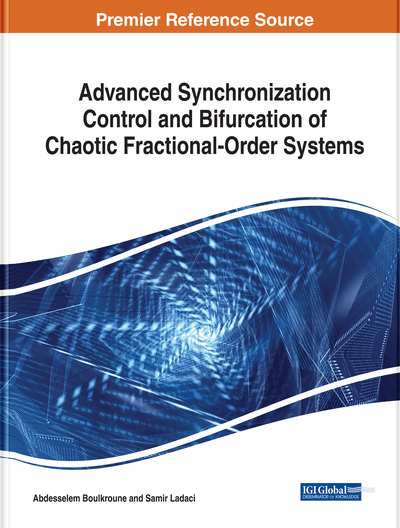# Backstepping Control for Synchronizing Fractional-Order Chaotic Systems

Amina Boubellouta (University of Jijel, Algeria)
Copyright: © 2018 |Pages: 37
DOI: 10.4018/978-1-5225-5418-9.ch005

## Abstract

In this chapter, one develops a fuzzy adaptive backstepping control-based projective synchronization scheme of a class of uncertain fractional-order nonlinear systems with unknown external disturbances. In each step, an uncertain nonlinear function is online modeled via a fuzzy logic system, and a virtual control term is determined based on the fractional Lyapunov stability. At the final step, a fuzzy adaptive control law ensuring the convergence of the projective synchronization error as well as the stability of the closed-loop control system is derived. Numerical simulations given at the end of this chapter confirm well the effectiveness of the proposed control method.
Chapter Preview
Top

## Introduction

In the last four decades, fractional calculus has attracted much attention from physicists and engineers owing to its attractive properties and potential applications, for reviews in this topic one can refer to Podlubny (1999), Shen & Lam (2014), Aguila-Camacho et al. (2014), Duarte-Mermoud et al. (2015), Liu et al. (2017a), Bao & Cao, (2015), Bao et al. (2015), Liu et al. (2017), Geng et al. (2016), Bouzeriba et al. (2015), Bouzeriba et al. (2016a), Boulkroune et al. (2015a), and Boubellouta & Boulkroune (2016). It has been proved that the fractional-order differential equation is an effective means for a precise modeling of the nonlinear and complex dynamics of many physical systems and some particular materials (Podlubny, 1999; Shen & Lam, 2014; Aguila-Camacho et al., 2014; Duarte-Mermoud et al., 2015; Liu et al., 2017a; Bao & Cao, 2015). The fractional-order differential equation is considered as a natural generalization of the classical integer-order differential equation. For more information about the fractional calculus, for instance one can refer to Liu et al. (2015a), Liu et al. (2015b), Wen et al. (2016), Yu et al. (2017). Numerous attractive fundamental results have been recently reported, in the literature, on the stability analysis and control design problems of fractional-order systems. For instance, a novel fractional-order Lyapunov stability method has been proposed for a class of fractional-order nonlinear dynamic systems in Li et al. (2009). A robust nonlinear fractional-order state observer has been designed in Boroujeni & Momeni (2012) for a class of nonlinear fractional-order systems. In Yang & Chen (2015), a finite-time stabilization approach for impulsive switched systems with fractional-order dynamics has been proposed. In Liu et al. (2015a), Liu et al. (2015b), and Liu et al. (2015c), an adaptive control methodology has been used also to effectively stabilize a class of uncertain fractional-order systems. Some intelligent (fuzzy or neural networks) feedback control approaches for uncertain fractional-order chaotic systems have been proposed in Huang et al. (2014), Boulkroune et al. (2016a), and Bouzeriba et al. (2016b; 2016c). Considering this important number of works related to fractional-order (linear or nonlinear) systems, it is very clear that its stability analysis and their control design problems are becoming hot research areas.

## Complete Chapter List

Search this Book:
Reset